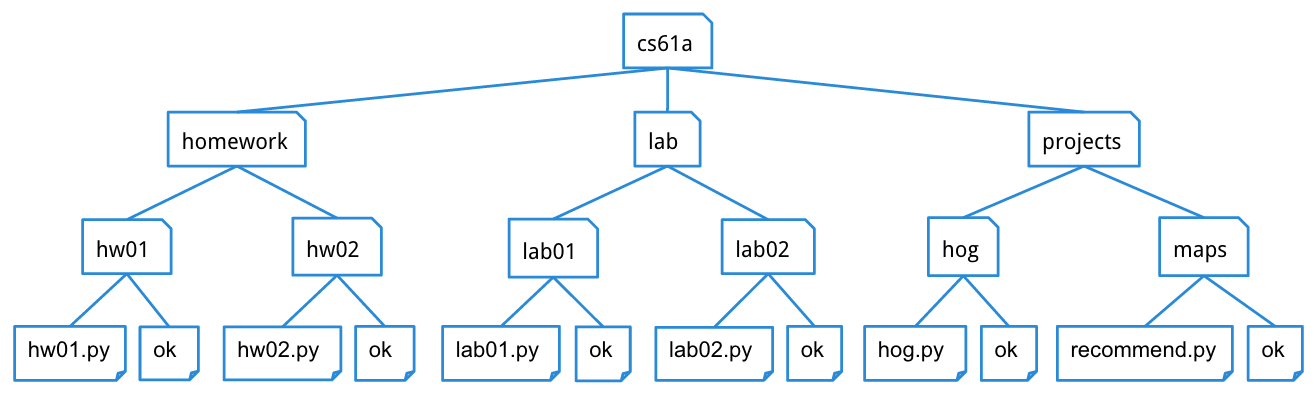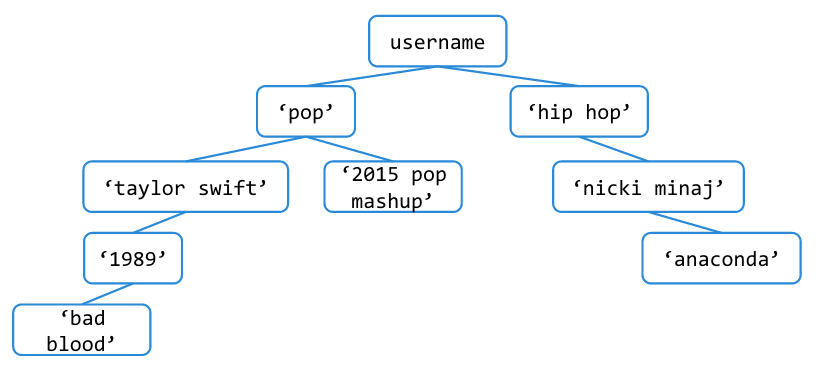Due at 11:59pm on 07/14/2015.

## Starter Files

Download lab07.zip. Inside the archive, you will find starter files for the questions in this lab, along with a copy of the OK autograder.

## Submission

By the end of this lab, you should have submitted the lab with `python3 ok --submit`. You may submit more than once before the deadline; only the final submission will be graded.

• To receive credit for this lab, you must complete Questions 1, 2, 3, and 4 in lab07.py and submit through OK.
• Questions 5 is extra practice. It can be found in the lab07_extra.py file. It is recommended that you complete this problem on your own time.

## Trees

A `tree` is a data structure that represents a hierarchy of information. A file system is a good example of a tree structure. For example, within your `cs61a` folder, you have folders separating your `projects`, `lab` assignments, and `homework`. The next level is folders that separate different assignments, `hw01`, `lab01`, `hog`, etc., and inside those are the files themselves, including the starter files and `ok`. Below is an incomplete diagram of what your `cs61a` directory might look like.As you can see, unlike trees in nature, which is where this data structure gets its name from, CS trees are drawn with the root at the top and the leaves at the bottom.

• node: a single unit in a tree.
• entry: the data inside of each tree's root node.
• root: the node at the top of a tree. every tree has one root node.
• subtree: a tree within the main tree, has its own entry and subtrees.
• branch: connects two nodes.
• leaf: a node that has no subtrees.

Our `tree` abstract data type consists of the `entry` at its root node and a list of its `subtrees`. To create a tree and access its entry and subtrees, use the following constructor and selectors:

• Constructor

• `tree(entry, subtrees=[])`: creates a tree object with the given `entry` and list of `subtrees`.
• Selectors

• `entry(tree)`: returns the value at the root of the `tree`.
• `subtrees(tree)`: returns the list of subtrees of the given `tree`.
• `is_leaf(tree)`: returns `True` if `tree`'s list of `subtrees` is empty, and `False` otherwise.

For example, the tree generated by

``````t = tree(1, [tree(2),
tree(3, [tree(4), tree(5)]),
tree(6, [tree(7)])])``````

would look like this:

``````   1
/ | \
2  3  6
/ \  \
4   5  7``````

It may be easier to visualize this translation by formatting the code like this:

``````t = tree(1,
[tree(2),
tree(3,
[tree(4),
tree(5)]),
tree(6,
[tree(7)])])``````

To extract the number `3` from this tree, which is the entry of its second subtree, we would do this:

``entry(subtrees(t))``

The `print_tree` function prints out a tree in a human-readable form. The exact form follows the pattern illustrated above, where the root is unindented, and each of its subtrees is indented one level further.

``````def print_tree(t, indent=0):
"""Print a representation of this tree in which each node is
indented by two spaces times its depth from the entry.

>>> print_tree(tree(1))
1
>>> print_tree(tree(1, [tree(2)]))
1
2
>>> print_tree(numbers)
1
2
3
4
5
6
7
"""
print('  ' * indent + str(entry(t)))
for subtree in subtrees(t):
print_tree(subtree, indent + 1)``````

## pyTunes

The CS 61A staff has created a music library called pyTunes. pyTunes organizes songs in folders that are labeled by category — in other words, in a tree! The `entry` at the root of the tree is your account name, which branches out into a hierarchy of categories: genres, artists, and albums, in that order. Songs (leaves in the tree) can be stored at any of these levels. A category cannot be empty (i.e. there will never be a node for a genre, artist, or album with no subtrees).

### Question 1: Create pyTunes

All pyTunes accounts come with the free songs below. Define the function `make_pytunes`, which takes in `username` and creates this tree:The doctest below shows the `print_tree` representation of a default pyTunes tree.

``````def make_pytunes(username):
"""Return a pyTunes tree as shown in the diagram.

>>> pytunes = make_pytunes('i_love_t_swift')
>>> print_tree(pytunes)
i_love_t_swift
pop
taylor swift
1989
2015 pop mashup
hip hop
nicki minaj
anaconda
"""
[tree('pop',
[tree('taylor swift',
[tree('1989',
tree('2015 pop mashup')]),
tree('hip hop',
[tree('nicki minaj',
[tree('anaconda')])])])``````

Use OK to test your code:

``python3 ok -q make_pytunes``

### Question 2: Number of Songs

A pyPod can only hold 10 songs, and you need to find out whether or not all the songs in your pyTunes account will fit. Define the function `num_songs`, which takes in a pyTunes tree `t` and returns the number of songs in `t`. Recall that there are no empty directories in pyTunes, so all leaves in `t` are songs.

Hint: You can use `is_leaf` to check whether a given tree is a leaf.

``````>>> no_subtrees = tree(1)
>>> is_leaf(no_subtrees)
True
>>> is_leaf(tree(5, [tree(3), tree(4)]))
False``````
``````def num_songs(t):
"""Return the number of songs in the pyTunes tree.

>>> pytunes = make_pytunes('i_love_t_swift')
>>> num_songs(pytunes)
3
"""
if (is_leaf(t)):
return 1
return sum([num_songs(s) for s in subtrees(t)])

# Alternate solution
def num_songs(t):
if is_leaf(t):
return 1
leaves = 0
for subtree in subtrees(t):
leaves += num_songs(subtree)
return leaves``````

Use OK to test your code:

``python3 ok -q num_songs``

### Question 3: Ctrl + F

In order to check if your pyTunes account contains a certain song or category, define the function `find`. It takes in a pyTunes tree `t` and returns `True` if `t` contains a either a song or a category called `target` and `False` otherwise.

``````def find(t, target):
"""Returns True if t contains a node with the value 'target' and False otherwise.

>>> my_account = tree('kpop_king',
...                    [tree('korean',
...                          [tree('gangnam style'),
...                           tree('wedding dress')]),
...                     tree('pop',
...                           [tree('t-swift',
...                                [tree('blank space')]),
...                           tree('uptownfunk')])])
>>> find(my_account, 'korean')
True
>>> find(my_account, 'blank space')
True
False
"""
if entry(t) == target:
return True
if is_leaf(t):
return False
return any([find(s, target) for s in subtrees(t)])

# Alternate solution
def find(t, target):
if entry(t) == target:
return True
for subtree in subtrees(t):
if find(subtree, target):
return True
return False``````

Use OK to test your code:

``python3 ok -q find``

### Question 4: Delete

You also want to be able to delete a song or category from your pyTunes. Define the function `delete`, which takes in a pyTunes tree `t` and returns a new tree that is the same as `t` except with `target` deleted. If `target` is a genre, artist, or album, delete everything inside of it. It should not be possible to delete the entire account or `root` of the tree.

``````def delete(t, target):
"""Returns the tree that results from deleting target from t. If target is a category,
delete everything inside of it.

>>> my_account = tree('kpop_king',
...                    [tree('korean',
...                          [tree('gangnam style'),
...                           tree('wedding dress')]),
...                     tree('pop',
...                           [tree('t-swift',
...                                [tree('blank space')]),
...                           tree('uptownfunk')])])
>>> new = delete(my_account, 'pop')
>>> print_tree(new)
kpop_king
korean
gangnam style
wedding dress
"""
kept_subtrees = []
for subtree in subtrees(t):
if entry(subtree) != target:
kept_subtrees += [delete(subtree, target)]
return tree(entry(t), kept_subtrees)

# Alternate solution
def delete(t, target):
kept_subtrees = [delete(sub, target) for sub in subtrees(t) if entry(sub) != target]
return tree(entry(t), kept_subtrees)``````

Use OK to test your code:

``python3 ok -q delete``

## Extra Questions

The following questions are for extra practice — they can be found in the the lab07_extra.py file. It is recommended that you complete these problems on your own time.

Of course, you should be able to add music to your pyTunes. Write `add_song` to add `song` to the given `category`. You should not be able to add a song under a song or to a category that doesn't exist. See the doctests for examples.

``````def add_song(t, song, category):
""" Returns a new tree with song added to category. Assume the category

>>> indie_tunes = tree('indie_tunes',
...                  [tree('indie',
...                    [tree('vance joy',
...                       [tree('riptide')])])])
>>> new_indie = add_song(indie_tunes, 'georgia', 'vance joy')
>>> print_tree(new_indie)
indie_tunes
indie
vance joy
riptide
georgia

"""
if entry(t) == category:
return tree(entry(t), subtrees(t) + [tree(song)])
kept_subtrees = []
for sub in subtrees(t):
``python3 ok -q add_song``